GNSS Geodesy Innovation Interviews UAV/UAS Positioning Surveying Applications Perspective Time Navigation Mapping Policy Land Administration News Archives LBS GIS SDI Imaging E-Zine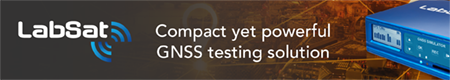GNSS

# Simulation of Galileo E5 Signal

May 2008 | Comments Off on Simulation of Galileo E5 Signal

Fantinato Samuele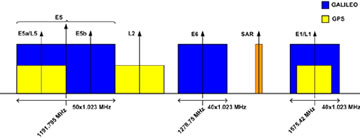Figure 1 Galileo spectrum allocation

E5 band (1164 -1215 MHz), composed of E5a and E5b bands, is part of the spectrum allocated by ITU for new Radio Navigation Satellite Services in 2000. E5 signal has the wider bandwidth (51.150 MHz) never used in satellite navigation. Galileo E5 signal is composed by two data components and two pilot components broadcasted together by means of the multiplexing scheme AltBOC(15,10). E5a band will be used for Freely/NAV message (Open Service) and the codes of data and pilot components are uncripted, E5b band will be used for Integrity/NAV message for Safety of Life and Open Service. Integrity of signal is probably the most advanced service introduced by Galileo . One of the main feature of E5 band is that the signal can be received in two ways: the first is to filter and demodulate only one of side bands E5a or E5b (see Fig. 1) the second is to process the overall received signal containing the components of both bands ,. In this sections is considered the second way because it will be adopted by professional receivers and this will avoid to obtain all the advantages of Galileo signal. E5 is the most promising signal in terms of performance in multipath environment and positioning for critical applications but also the most challenging for a receiver or a simulation. In this paper will be presented the simulation of the transmission and reception of E5 signal. It will be first described the generation of codes, then it will be illustrated Galileo AltBOC(15,10) signal structure and its differences with a conventional AltBOC, it will be shown a way for the generation of that signal and some basic characteristics for the development of a software receiver for E5.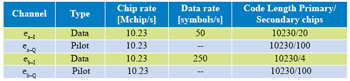Table 1 Feature of E5 components

## Simulation

The simulation is developed using Matlab. Four satellites are transmitting Galileo AltBOC(15,10) signal in steps of one millisecond.
For each transmitted signal some effects are introduced like doppler shift due to relative motion between satellite and receiver, delay, multipath channel and gaussian noise and then the overall received signal is processed with some algorithms typical of software receivers. All the blocks are simulated at baseband.

## Signal Generation

The first step in the simulation is the generation of Galileo E5 codes. In E5 signal there are four components or channels: , , and . Each component has its own code which is the product of the repetition of a primary code of 10230 chips, corresponding to 1 ms of signal, and a secondary code of different length, see Tab. 1. “Galileo Signal In Space Interface Control Document” (SIS ICD) gives the basis for generation of codes: the primary codes are generated by means of two Linear Feedback Shift Registers. For each satellite SIS ICD gives the “start values” and the values of feedback taps as polynomials in octal notation and some instructions to build correctly the LFSR. The secondary codes are only the conversion in binary representation of a hexadecimal number. Through some Matlab functions primary and secondary codes are generated for all satellites involved in simulation. As known in a Matlab simulation is necessary to define the reference time domain of each signal. The bits of the codes are then mapped in +1 BPSK values producing the discrete signals ex–y(nTc) with Tc 97.75ns that is the chip period. and are data channels with data rate reported in Tab. 1, and so the ranging codes are multiplied by two different data streams, and are pilot channels containing only the ranging codes. In conventional AltBOC the complex signal eb (t) = eb–I (t) + jeb–Q (t) is multiplied by a complex squared subcarrier er (t) = cr (t) + jsr (t) where cr (t) is the sign of a cosine function and Sr (t) is the sign of a sine function and the complex signal ea (t) = ea–I (t) + jea–Q (t) is multiplied by the complex conjugate of er (t), i.e. er *(t) obtaining the signal: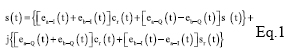In Figure 3 is represented how an AltBOC signal is built and its power spectral density in which one can recognize the two main lobes that represent the bands E5a and E5b. The Galileo AltBOC(15,10) signal structure is more complex than conventional AltBOC and has expression: where: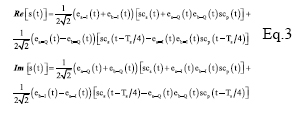where SCS(t) and SCp(t) are two subcarriers quantized in four values (Fig. 4).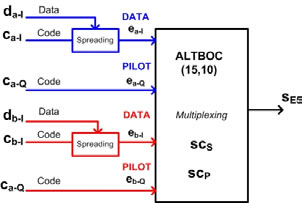Figure 2 Generation of AltBOC(15,10) signal

–~~~~~~~~~~~~–

Fantinato Samuele

In Galileo AltBOC has been modified introducing two different subcarriers and the products of the components in order to obtain a signal that lies on an 8-PSK constellation (constant envelope) which is very important for satellites high power amplifiers.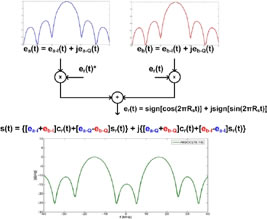Figure 3 Construction and Power Spectral Density of AltBOC Signal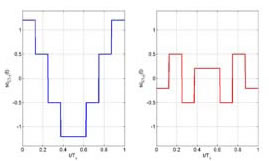Figure 5 Real and Imaginary part of E5 ideal signal, E5 filtered signal and its samples (K=8)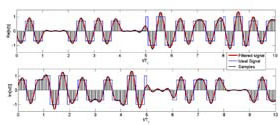Figure 5 Real and Imaginary part of E5 ideal signal, E5 filtered signal and its samples (K=8)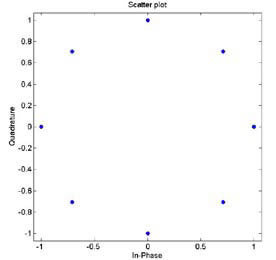Figure 6 Constellation of E5 signal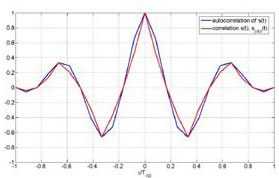Figure 7 Shapes of autocorrelation of S(t) and correlation between S(t) and Spilot(t)

In the following lines is reported how, in the simulation, the samples of E5 signal are generated, for more details see . As known in AltBOC(n, m), n represent the chip rate of the signal and m the frequency of the subcarriers. As one can see the subcarrier period is Ts = (n/m) Tc = (2/3)Tc. The two subcarriers are defined every Ts/8, so after the multiplexing scheme each chip period is composed of N = 12 slots in which the values of the signal can be different. The components ex– Y(nTc) are then interpolated with a “hold interpolator” of factor N = 12 and so directly multiplexed according to the expression of Eq. 2. The output signal is defined in TAB = Tc/12.
Now is shown the relationship between the chip rate Rc = 1/Tc and the bandwidth in which the signal should be transmitted. The one side bandwidth is B = 25.575MHz that B = 2.5Rc. Now is necessary to select a correct sampling frequency: Fsamp = KB with K > 2. So the ratio between the sample time, Tsamp = 1/Fsamp, and TAB is Tsamp/TAB = 24/5K. For example if K = 4 one have to upsample the signal by a factor 5, filter (In the simulation is introduced a raised root cosine filter with roll-off factor a =0.22) it in order to bandlimiting it, and then sample it with a factor 6 obtaining 10 samples for chip. These equations and values can be important in the development of a software receiver for E5, in particular the value of K determine the sampling frequency and so the resolution of the receiver. The procedure illustrated can be useful in a software receiver for the efficient generation of the local signals for the correlations with the input signal. Real and imaginary part of AltBOC(15,10) signal are in Fig. 5 and its constellation is represented in Fig. 6.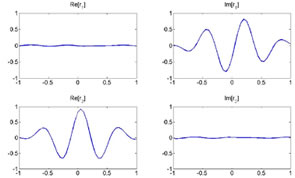Figure 8 Shapes of correlations r1(t) and r2(t)

## Acquisition, Tracking and Data Recovery

As previously said it has been developed a receiver for the demodulation of the overall E5 signal: the signal in input at the receiver is directly sampled (in a simulation the signal is already sampled) and the complex samples are then processed. In the acquisition and tracking process is performed a correlation between the received sampled signal and the samples of a local AltBOC(15,10) pilot signal defined as: Eq.4 This signal can been obtained from Eq. 2 setting to zero the data components ea–I and eb–I and the different normalization factor is introduced in order to not reduce the peak of correlation. In figure 7 one can observe that there is no a significant difference between the autocorrelation of S(t) signal and the correlation between S(t) and Spilot(t). The acquisition is performed using FFT based technique  and the delay and doppler frequency are estimated with precision depending on the sample time Tsamp and on the length of correlation. Also for the tracking of the signal the correlation is between the received signal and pilot signal of Eq. 4. For the tracking it is implemented a non-coherent Early minus Late DLL .
The data recovery operations use two local sampled signals (Eq. 5 and 6) that are both correlated with the incoming received signal . These signal are obtained setting to zero the pilot components in S(t). This choice is made because using only one signal (Sdata–1(t)) shows a problem when the sign of the two data is opposite: there is a zero in the middle of both real and imaginary part of correlation
Making correlation with these two signals for the decision for the transmitted data is necessary to observe only real parts of the two correlations. For example when the data of component ea–I = –1 nd the data of component eb–I = 1 the shapes of real and imaginary parts of correlations r1(t) and r2(t) between the received signal and Sdata– 1(t) and Sdata–2(t) respectively are in Fig. 8. In the table 2 are summarized all the cases.

## Conclusion

In this paper have been described the main features of Galileo E5 signal and some algorithms for signal generation and software receivers. In particular have been highlighted differences between conventional AltBOC and that used in Galileo: two subcarriers are used and the signal has an 8-PSK constellation. Have been given some useful equations for the implementation of a signal generator or the development of a simulation and have been proposed some techniques for the acquisition, tracking and data recovery of the overall received signal.

## References

 ESA,GJU “Galileo Open Service: Signal In Space Interface Control Document (OS SIS ICD) Draft 0’’, 23-05-2006
 G. W. Hein, J. Godet, J.L. Issler, J.C. Martin, P. Erhard, R. L.Rodriguez, T. Pratt “Status of Galileo Frequency and Signal Design’’, Brussels , 2002
 L. Ries, L. Lestarquit, E.A. Miret, F. Legrand, W. Vigneau, C. Bourga “A Software Simulation Tool for GNSS2 BOC Signals Analysis’’, 2002
 D.J.R. Van Nee, A.J.R.M. Coenen “New Fast GPS Code- Acquisition Tecnique Using FFT’’, ELECTRONICS LETTERS 17th January 1991 Vol. 27 No. 2
 K. Krumvieda, P. Madhani, C. Cloman, E. Olson, J. Thomas, P. Axelrad, W. Kober “A Complete IF Software GPS Receiver: A Tutorial about the Details’’, ION GPS 2001, Salt Lake City, 2001
 W. Zhuang, J. Tranquilla “Modelling and Analysis for the GPS Pseudo- Range Observable’’, IEEE TRANSACTIONS ON AEROSPACE AND ELECTRONIC SYSTEMS VOL. 31, NO. 2 APRIL 1995
 J.M. Sleewaegen, W. De Wilde, M. Hollreiser “Galileo AltBOC Receiver’’, 2004
 N. Gerein, A. Manz, M. Clayton, M. Olynik “Galileo Sensor Station Ground Reference Receiver Performance Characteristics’’, ION GPS/GNSS 2003, 9-12 Sept 2003, Portland, OR
 N.Gerein “Hardware Architecture for Processing Galileo Alternate Binary Offset Carrier (AltBOC) signals’’, United States Patent, No. 6,922,177 B2, July 2006
 M.C. Jeruchim, P. Balaban, K.S. Shanmugan “Simulation of Communication Systems’’, Plenum Publishing Corporation, New York, 1992
 S. Fantinato, S. Pupolin, L. Vangelista “Analysis of Galileo AltBOC(15,10) Signal for Simulations and Software Receivers’’, Proceedings of WPMC 2007, Jaipur (India)
 S. Fantinato, Thesis: “Development of a software receiver for Galileo system’’, Padova, 2007Fantinato Samuele Fantinato Samuele: he got a degree in Telecommunications Engineering in July 2007 at University of Padova, Italy, with the top of the marks. He was at “Department of Information Engineering’’. He joined Qascom Srl (an Italian company working in GPS security and authentication), during the thesis period. He is now interested in working in companies researching in navigation and satellite communication systems. samuele.fantinato@gmail.com

##### INDUSTRY | LBS | GPS | GIS  | REMOTE SENSING | GALILEO UPDATE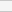(No Ratings Yet)Loading...